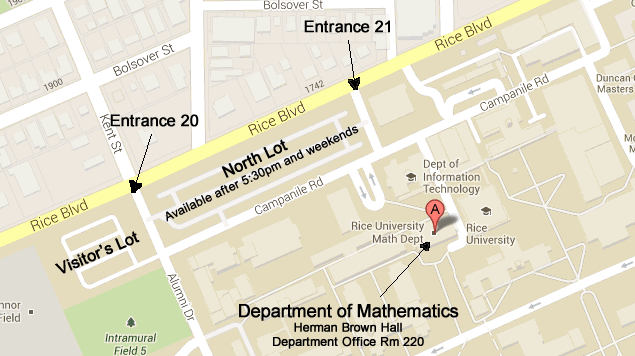## Mathematical physics

### Faculty

• David Damanik

Spectral theory, mathematical physics, and analysis.

• Robert Hardt (Emeritus)

Geometric measure theory, partial differential equations, continuum mechanics.

• Milivoje Lukic

Schröedinger operators and nonlinear integrable partial differential equations.

• Christos Mantoulidis

Differential geometry, Partial differential equations, Mathematical general relativity, Geometric measure theory, and Geometric flows.Large Map

Tel (713) 348-4829